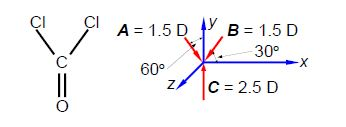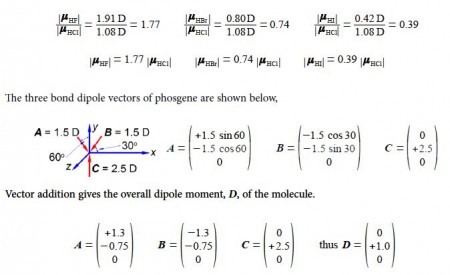# Sample Question: Hydrogen Halide Dipole Moments

The magnitudes of the four hydrogen halide dipoles are given below.Firstly, using vector notation, express the magnitudes of the HF, HBr and the HI dipoles as scalar multiples of the HCI dipole magnitude. Secondly, below Figure shows phosgene, in what direction does the phosgene molecular dipole point and what is its magnitude assuming the bond angles are 120° apart?Solution

The ratios of the magnitudes are and thus the scalar multiples of the HO dipole magnitude areThe horizontal components for each of the two C-Cl bond dipoles cancel one another out. The molecular dipole of phosgene is 1.0 D along the y-axis in the positive direction.